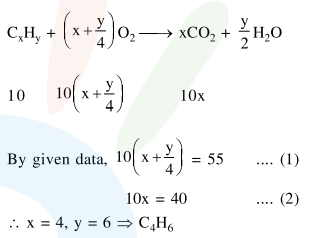Deepak Scored 45->99%ile with Bounce Back Crack Course. You can do it too!

# Solve this following

Question:

At $300 \mathrm{~K}$ and 1 atmospheric pressure, $10 \mathrm{~mL}$ of a hydrocarbon required $55 \mathrm{~mL}$ of $\mathrm{O}_{2}$ for complete combustion and $40 \mathrm{~mL}$ of $\mathrm{CO}_{2}$ is formed. The formula of the hydrocarbon is:

1. $\mathrm{C}_{4} \mathrm{H}_{8}$

2. $\mathrm{C}_{4} \mathrm{H}_{7} \mathrm{Cl}$

3. $\mathrm{C}_{4} \mathrm{H}_{10}$

4. $\mathrm{C}_{4} \mathrm{H}_{6}$

Correct Option: , 4

Solution: